# 2nd PUC Physics Question Bank Chapter 15 Communication Systems

## 2nd PUC Physics Question Bank Chapter 15 Communication Systems

### 2nd PUC Physics Chapter 15 Communication Systems NCERT Text Book Questions and Answers

Question 1.
Which of the following frequencies will be suitable for beyond the horizon communication using sky waves?
(a) 10 kHz
(b) 10 MHz
(c) 1 GHz
(d) 1000 GHz
At frequencies above 40 MHz, the communication is limited to line of sight paths. Hence, the options (a) and (b) are correct.Question 2.
Frequencies in the UHF range normally propagate by means of:
(a) Ground waves.
(b) Sky waves.
(c) Surface waves.
(d) Space waves.
In the UHF range, the transmission is done by means of space waves. Hence, the option (d) is correct.

Question 3.
Digital signals
(i) do not provide a continuous set of values,
(ii) represent values as discrete steps,
(iii) can utilize a binary system, and
(iv) can utilize decimal as well as binary systems.

Which of the above statements are true?
(a) (i) and (ii) only
(b) (ii) and (iii) only
(c) (i), (ii) and (iii) but not (iv)
(d) All of (i), (ii), (iii) and (iv).
For digital signals, all the four options are true. Hence the options (d) is correct.

Question 4.
Is it necessary for a transmitting antenna to be at the same height as that of the receiving antenna for line-of-sight communication? A TV transmitting antenna is 81m tall. How much service area can it cover if the receiving antenna is at the ground level?
For line of sight communication, the receiving antenna must intercept the transmitted signal. If the signal is to be received. beyond horizon, the receiving antenna must be high enough to intercept the transmitted signal. Therefore, it is not necessary for a transmitting antenna to be at the same high as that of the receiving antenna.
Here, h = 81m; R = 6.4 x 106 m If d is the radius of the circle within which the transmission can be observed, then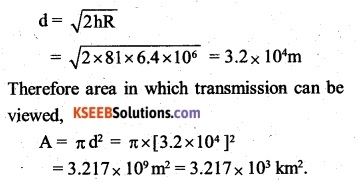Question 5.
A carrier wave of peak voltage 12V is used to transmit a message signal. What should be the peak voltage of the modulating signal in order to have a modulation index of 75%?
Here, Ec = 12V, ma = 75% = 0.75
Now, modulation factor,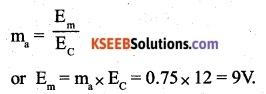Question 6.
A modulating signal is a square wave, as shown in Fin. 15.14.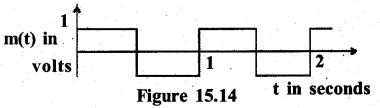The carrier wave is given by c (t) = 2sin (8 π t) volts.
(i) Sketch – the amplitude modulated waveform
(i) Here, c (t) = 2 sin 8 n t
It follows that the carrier is a sine wave having a period of T = 2π/8π= 0.25 s and
amplitude of EC=±2V
On the other hand, the modulating signal is a square wave of period 1 s and amplitude of E = ±1V.
Therefore, the maximum and minimum amplitude of the modulated waveform will be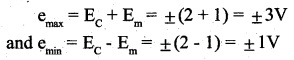Hence the amplitude modulated waveform will be as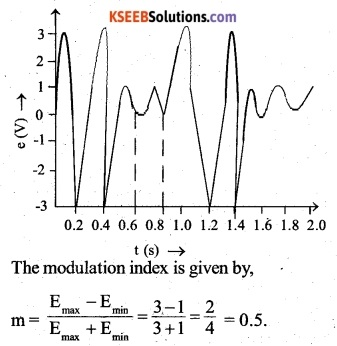Question 7.
For an amplitude modulated wave, the maximum amplitude is found to be 10V while the minimum amplitude is found to be 2V. Determine the modulation index μ.
What would be the value of p if the minimum amplitude is zero volt?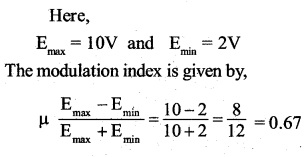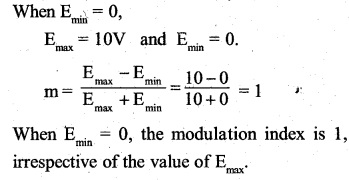Question 8.
Due to economic reasons, only the upper sideband of an AM wave is transmitted, but at the receiving station, there is a facility for generating the carrier. Show that if a device is available which can multiply two signals, then it is possible to recover the modulating signal at the receiver station.
When only the upper side band (we + wm) is transmitted, the transmitted signal may be represented as
e = Et cos (we + wm )t   ………………(1)
where is the amplitude of the transmitted signal Let the carrier wave available at the receiving station be
e =Ec cos wct  ……………(2)
where,
Ec is the amplitude of the carrier wave Multiplying the respective sides of equation (1) and (2).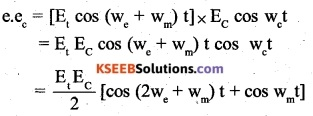If this signal is passed through a filter circuit, which acts as a block for the higher frequency (2we + wm) and allows the low frequency to pass through it, then the modulating signal given by,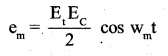can be recovered at the receiving station

### 2nd PUC Physics Chapter 15 Communication Systems Additional Questions and Answers

Question 1.
The audio signal can not be transmitted directly into the space. Why?

• The length of the antenna required is so large (500m) that is practically impossible to set up it.
• The energy radiated from the antenna in ‘ audio frequency range is practically zero
• The audio signals transmitted from the different broadcasting stations get inseparably mixed up.

Question 2.
600 Hz modulating voltage fed into FM generator produces a frequency deviation of 3.36 kHz. Find the modulation index.
Modulating frequency, FM = 600 Hz Frequency deviation,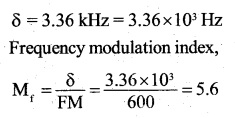Question 3.
Why is FM signal less susceptible to noise than an AM signal?
In FM transmission, the message signal is in the form of frequency variations of carrier waves. During modulation process, the noise gets amplitude modulated. Since it brings about variations only in the amplitude of carrier waves, it does no harm to the message signal. For this reason, FM signal is less susceptible to noise than an AM signal.

Question 4.
Ozone layer is present in
(A) Troposphere
(B) stratosphere
(C) Ionosphere
(D) Mesosphere
(B) stratosphereQuestion 5.
What is the cause of green house effect?
(A) IR rays
(B) UV rays
(C) x-rays
(A) IR rays

Question 6.
Communication on ground is through electromagnetic waves of wavelength
(A) Larger than 600m
(b) Between 200 and 600m
(C) Between 1 and 5m
(D) Between 10-13 and 0.1m
(D) Between 10-13 and 0.1m

Question 7.
The maximum distance upto which TV transmission from a TV tower of height h can be received is proportional to
(A) h1/2
(B) h3/2
(C) h
(D) h2
(D) h2

Question 8.
The output from a LASER is monochromatic. It means that it is
(A) Directional
(B) Polarised
(C) Narrow beam
(D) Single frequency
(D) Single frequencyQuestion 9.
Losses in optical fibre is caused by
(A) Impurities in glass
(B) Imperfect transparency of the glass
(C) Its extremely small area of cross section
(D) Stepped index structure